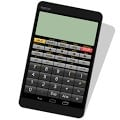# Panecal Scientific Calculator APK

A Scientific calculator, the display of which indicates expressions and gives easy operation for editing.
The Panecal scientific calculator includes features below:
*Arithmetic operations, trigonometric, inverse trigonometric, exponential, logarithmic functions, and so on
*Calculations with parentheses
*BIN, OCT, DEC, HEX and n-base number conversion
*Memory, M+, M-, MR, MC
*4 types of format mode: FloatPt, Fix, Sci, and Eng
*Scroll up/down lines
*Easy editing by using a cursor operation
*Cut, copy, and paste
*Configurable grouping separator and decimal point
*Horizontal screen display
*Vibration and orange coloring keys as tapping

Four arithmetic operations:
1 + 2 – 3 × 4 ÷ 5 = 0.6

Root:
√( 2 × 2 ) = 2

Trigonometric functions:
sin(30) = 0.5
asin(0.5) = 30
(Depends on DRG settings)

Parentheses:
( 1 + 2 ) × ( 3 + 4 ) = 21
( 1 + 2 ) ( 3 + 4 ) ( 5 + 6 ) = 231

Percentages:
500 + 20% = 600
500 – 20% = 400
500 × 20% = 100
100 ÷ 500% = 20

Degree, Minute, Second
30°30 = 30.5
1°30+2°45 = 4°15 = 4.25 (conversion by tapping [°’”] key)

BIN, OCT, DEC, HEX and n-base number conversion
1111(BIN) → 0F(HEX) key operation:[CLR][BIN]1111[HEX]
Grouping separator and decimal point
123,456,789.1 + 0.02 = 123,456,789.12
123.456.789,1 + 0,02 = 123.456.789,12
(Depends on settings)

[Disclaimer]
APPSYS does not accept responsibility for any loss which may arise from reliance on the software or materials published on this site.

#### What’s New

Version 6.5.1 Date 2017.2.8
– Fixed possible crash on some devices.

Name
Panecal Scientific Calculator
Package
jp.ne.kutu.Panecal
Version
6.5.1
Size
2.78 MB
Installs
Developed By
Appsys